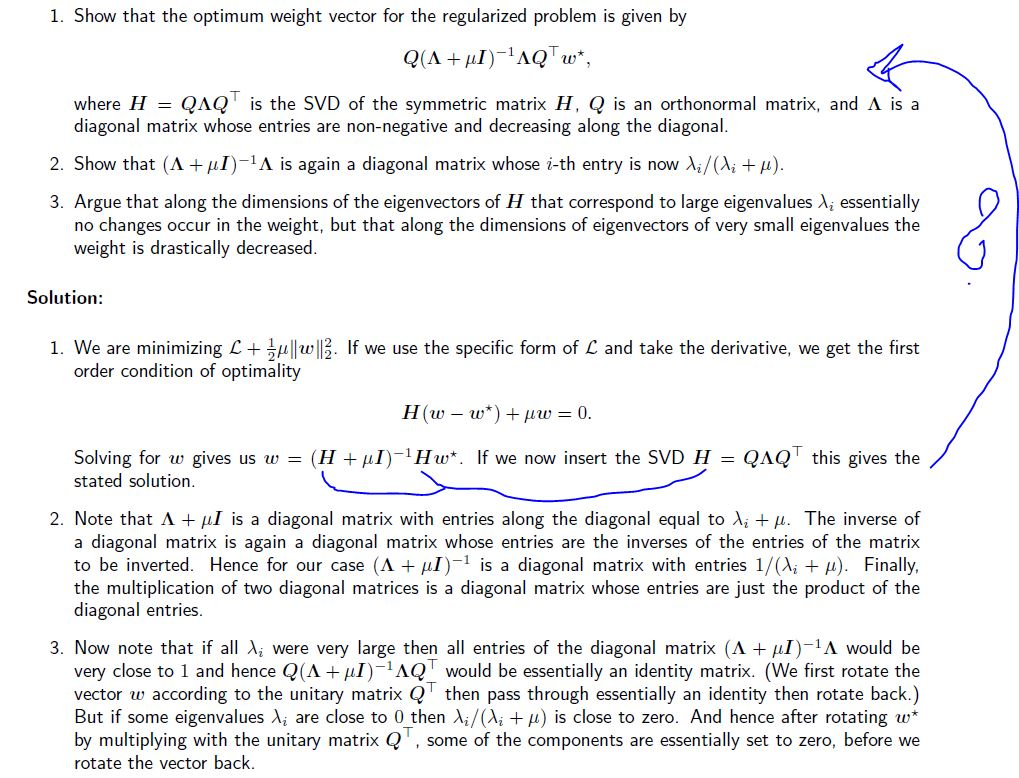### Lab 9 Problem 3

Hello,
in the solutions it says that inserting H = QLQ^T into w = (H + muI)^-1 H w* leads to the above solution,
but I dont see how

w = (QLQ^T - mu I )^-1 Q L Q^T w* reduces to that.

Thanks!Top comment

The essential question to ask is what is $$(H+\mu I)^{-1}$$. We only need to show that $$(Q\Lambda Q^{\top}+\mu I)^{-1}=Q(\Lambda+\mu I)^{-1}Q^{-1}$$, which is equivalent to show $$(Q\Lambda Q^{\top}+\mu I)Q(\Lambda+\mu I)^{-1}Q^{-1}=I$$, then it is straightforward to get the conclusion. Use the fact that Q is orthonormal
$$(Q\Lambda Q^{\top}+\mu I) Q(\Lambda+\mu I)^{-1}Q^{-1}=(Q\Lambda +\mu Q) (\Lambda+\mu I)^{-1}Q^{-1}=Q(\Lambda +\mu I) (\Lambda+\mu I)^{-1}Q^{-1}=QQ^{-1}=I$$.

Page 1 of 1